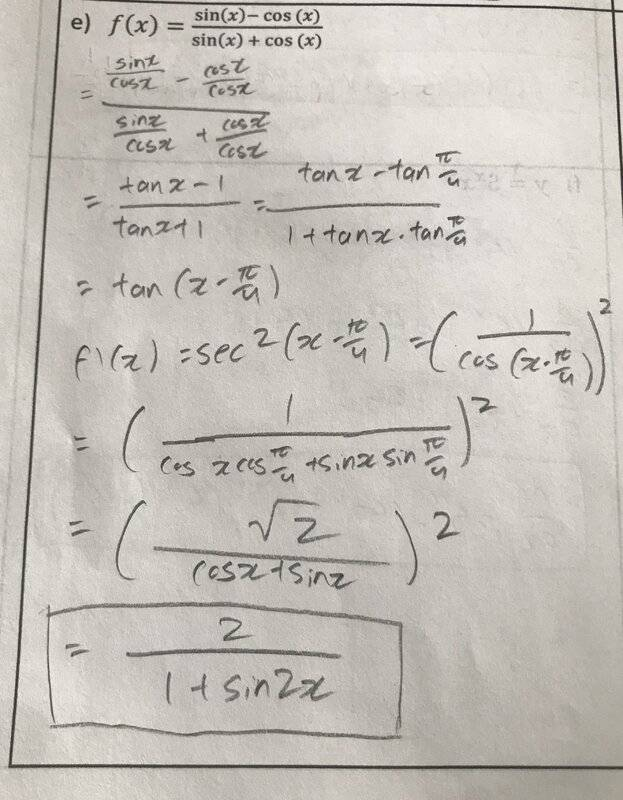# Differentiate, but do not simplify: ##f(x)=\frac{sin(x)-cos(x)}{sin(x)+cos(x)}##

Homework Statement:
I'm not sure if my latex is correct, but the image is provided with my work, please let me know if it's right!
Relevant Equations:
n/aehild
Homework Helper
Homework Statement:: I'm not sure if my latex is correct, but the image is provided with my work, please let me know if it's right!
Relevant Equations:: n/a

View attachment 262669
It is correct, but the solution is also simple if you differentiate the original function.
By the way, start and finish your latex formula with double \$:

$$f(x)=\frac{sin(x)-cos(x)}{sin(x)+cos(x)}$$
$$f(x)=\frac{sin(x)-cos(x)}{sin(x)+cos(x)}$$

Last edited:
•ttpp1124